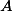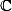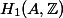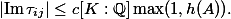# Martin Orr's Blog

## The matrix lemma for elliptic curves

Posted by Martin Orr on Friday, 25 May 2012 at 14:00

Letbe a principally polarised abelian variety of dimensionover. We can associate withacomplex matrix called the period matrix which roughly speaking describes a basis for the image ofin(actually it is not really the period matrix as it is only defined up to the action ofon the Siegel upper half space; we can make it nearly unique by forcing it to be in a particular fundamental domain).

The matrix lemma says that, ifis defined over a number field, then the entries of the imaginary part of the period matrix cannot be too large with respect to the height of(Faltings height or modular height).

Matrix lemma. (Masser 1987) Letbe a principally polarised abelian variety of dimensionover a number field. Letbe the period matrix forin the standard fundamental domain of the Siegel upper half space. There is a constantdepending only onsuch that all the entries ofsatisfyLast time I used a lower bound for the lengths of non-zero periods in the proof of the isogeny theorem. This follows from the matrix lemma as we can easily relate lengths of periods and the period matrix.

Today I will prove the matrix lemma for elliptic curves. The general proof requires various facts about Siegel modular forms and also uses a funny choice of level structure due to Igusa (I do not understand why). But the basic structure of the proof is already visible in the elliptic curves case and we can be concrete about the modular forms involved, using only facts I learned in Part III.